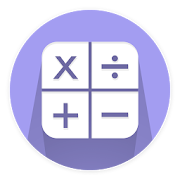# BODMAS CalculatorEveryone
31
What is BODMAS ?
BODMAS Stands for :

B stands for Brackets
O stands for Order
D stands for Division
M stands for Multiplication
S stands for Subtraction

So if we have to calculate 3 + 2 x 5 . We could either do a 3+2 first and then 5 x 5 or we could either do a 2×5 first and then 3 + 10 . How do we know what to follow ?

This is exactly where BODMAS came to picture . It simply means follow BODMAS when doing such calculations .

Answer to Above question could be either 25 or 13 . According to BODMAS it should be 13 because order of Multiplication comes first and then comes Addition so we multiply 2 with 5 first and then we add 3 with resulting 10 to give the final answer 13.

What is Calculator ?

By Calculator we mean a Simple Mathematical Calculator which calculates mathematical expressions and gives us result . For example 3+2 . We all know its 5 . But what about 232434 + 99238 ? Not that easy so we use a Calculator .

Again What is BODMAS Calculator ?

It is a Calculator which calculates mathematical operations following BODMAS Rule .

This is an android version of BODMAS Calculator .
Collapse

Review Policy
3.9
31 total
5
4
3
2
1

## What's New

Introduction of New Skin
power operator is added . So now you can calculate 2^3 = 8
Now can ad negative numbers in expression i.e. -2 X -5 is possible
operators can now be used in the starting of expression i.e. 0.2 X 0.4
Improved Look and Feel
Minor Bug Fixes
Collapse

Updated
November 11, 2018
Size
2.4M
Installs
5,000+
Current Version
1.5
Requires Android
4.0.3 and up
Content Rating
Everyone
Permissions
Offered By
VersionPB